# Search Results (8)

View
Selected filters:
• CCSS.Math.Content.3.NF.A.3d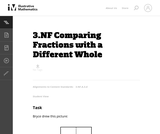Conditions of Use:
No Strings Attached
Rating

This task is meant to address a common error that students make, namely, that they represent fractions with different wholes when they need to compare them. This task is meant to generate classroom discussion related to comparing fractions. Particularly important is that students understand that when you compare fractions, you implicitly always have the same whole.

Subject:
Mathematics
Numbers and Operations
Material Type:
Activity/Lab
Provider:
Illustrative Mathematics
Provider Set:
Illustrative Mathematics
Author:
Illustrative Mathematics
08/10/2012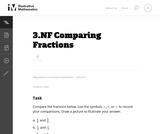Conditions of Use:
No Strings Attached
Rating

The purpose of this task is for students to compare fractions using common numerators and common denominators and to recognize equivalent fractions.

Subject:
Mathematics
Numbers and Operations
Material Type:
Activity/Lab
Provider:
Illustrative Mathematics
Provider Set:
Illustrative Mathematics
Author:
Illustrative Mathematics
08/15/2012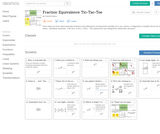Rating

Show what you know about equivalent fractions and ordering by choosing three activities (in a row, column, or diagonal) to complete the tic-tac-toe board. Standards assessed: 3.NF.3a-d, 4.NF.1, 4.NF.2. This assessment was designed for adult basic education.

Subject:
Mathematics
Numbers and Operations
Material Type:
Assessment
Interactive
Author:
Connie Rivera
11/10/2017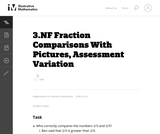Conditions of Use:
No Strings Attached
Rating

This is a task from the Illustrative Mathematics website that is one part of a complete illustration of the standard to which it is aligned. Each task has at least one solution and some commentary that addresses important asects of the task and its potential use. Here are the first few lines of the commentary for this task: Who correctly compares the numbers 2/3 and 2/5? Ben said that 2/3 is greater than 2/5. Lee said that 2/3 is equal to 2/5. Mia said that 2/3 is less tha...

Subject:
Mathematics
Material Type:
Activity/Lab
Provider:
Illustrative Mathematics
Provider Set:
Illustrative Mathematics
Author:
Illustrative Mathematics
03/18/2013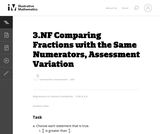Conditions of Use:
No Strings Attached
Rating

This is a task from the Illustrative Mathematics website that is one part of a complete illustration of the standard to which it is aligned. Each task has at least one solution and some commentary that addresses important asects of the task and its potential use. Here are the first few lines of the commentary for this task: Choose each statement that is true. $\frac98$ is greater than $\frac{9}{4}$. $\frac{9}{4}$ is greater than $\frac98$. $\frac98 \gt \frac{9}{4}$. \frac... Subject: Mathematics Material Type: Activity/Lab Provider: Illustrative Mathematics Provider Set: Illustrative Mathematics Author: Illustrative Mathematics Date Added: 03/18/2013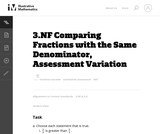Conditions of Use: No Strings Attached Rating This is a task from the Illustrative Mathematics website that is one part of a complete illustration of the standard to which it is aligned. Each task has at least one solution and some commentary that addresses important asects of the task and its potential use. Here are the first few lines of the commentary for this task: Choose each statement that is true.\frac34$is greater than$\frac54$.$\frac54$is greater than$\frac34$.$\frac34 \gt \frac54$.$\frac34 \lt \frac...

Subject:
Mathematics
Material Type:
Activity/Lab
Provider:
Illustrative Mathematics
Provider Set:
Illustrative Mathematics
Author:
Illustrative Mathematics
03/18/2013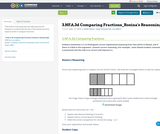Conditions of Use:
Remix and Share
Rating

This flawed reasoning task provides practice for students to understand the size of the wholes must be equal in order to compare fractions.

Subject:
Elementary Education
Mathematics
Material Type:
Activity/Lab
Assessment
Homework/Assignment
Author: